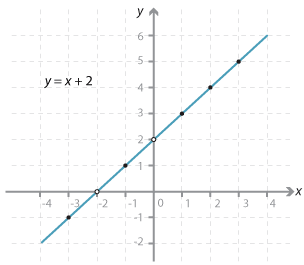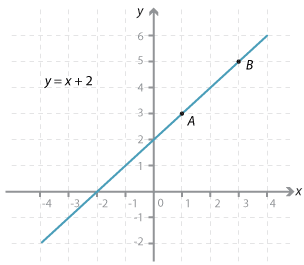### Graphing straight lines two-point method

Consider the line y = x + 2.

To graph it, we could draw a table of values, plot the points and join them.

 x y –3 –2 –1 0 1 2 3 –1 0 1 2 3 4 5

This line has gradient = 1, y-intercept = 2.Detailed description

Two points determine a straight line. This line could be draw just with two of the above points.

When x = 1, y = 3.

When x = 3, y = 3 + 2 = 5.

The points (1, 3) and (3, 5) are plotted and the line drawn through them.

The y-intercept is 2 and the gradient = 1.Detailed description